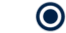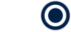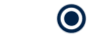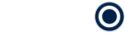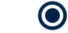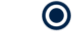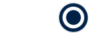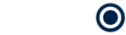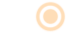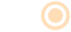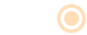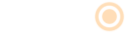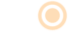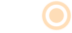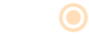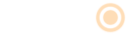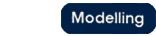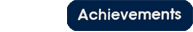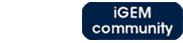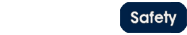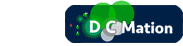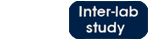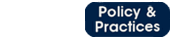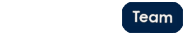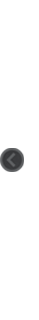# Team:Oxford/why do we need microcompartments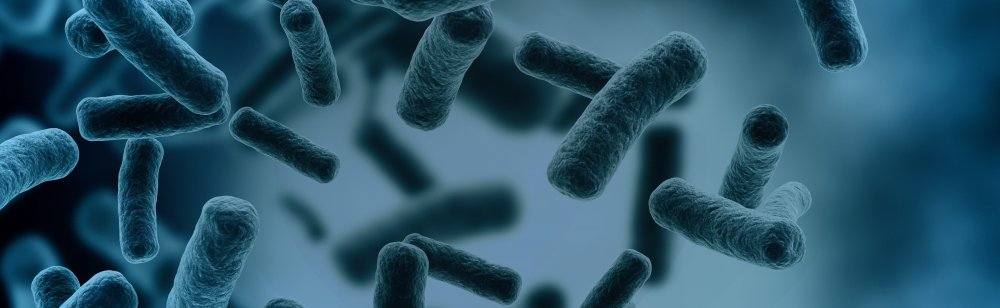Why are we using them?# Why do we need microcompartments?

Using microcompartments, we can boost the bioremediation capacity of E. coli and P. putida in two key ways: by increasing the degradation rate of DCM, and by preventing toxic metabolic intermediates from damaging the cell. How this happens and to what extent we expect this to improve DCMation is described below.

Increasing the reaction rate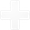Increasing the reaction rate

# Increased concentration of metabolic enzymes

Proteins can be targeted into microcompartments via an 18-amino acid leader sequence that forms a well-defined helical structure (Lawrence AD, 2014). We added this leader sequence to dcmA in both E. coli and P. putida. The resulting accumulation of dcmA in the microcompartments leads to an increased local concentration of the metabolic enzyme. This allows more rapid degradation of DCM, which can diffuse freely through the plasma membrane and into microcompartments.Oxford iGEM 2014

# Preventing interference with cell metabolism

Additionally, the products of DCM degradation are only produced within the confines of the microcompartment. This prevents HCl and formaldehyde from interfering with other metabolic processes in the cell.
Introduction to stochastic diffusion modelsIntroduction to stochastic diffusion models

# Stochastic Reaction-Diffusion models

Because of the relatively small number of molecules we are expecting to have in our cells (≈10^5 enzymes per cell and 10^3 enzymes per microcompartment), we developed stochastic reaction-diffusion models to predict the distribution of formaldehyde within the system. These stochastic models build in an element of randomness that reflects the nature of diffusion for systems with few elements in a way that deterministic relationships such as Fick’s law do not.

We approached this problem in a number of different ways. Initially, we built a system in which molecules would move a scaled random distance selected from a normal distribution at every time interval dt. This was adapted from the Smoluchowski equations which state:

X(t+ ∆t)=X(t)+ √2D∆t ε
Y(t+ ∆t)=Y(t)+ √2D∆t ε
Z(t+ ∆t)=Z(t)+ √2D∆t ε

• X(t),Y(t),Z(t) = particle co-ordinates at time t
• D = diffusion constant
• ε = normally distributed random variable
• ∆t = small time interval

•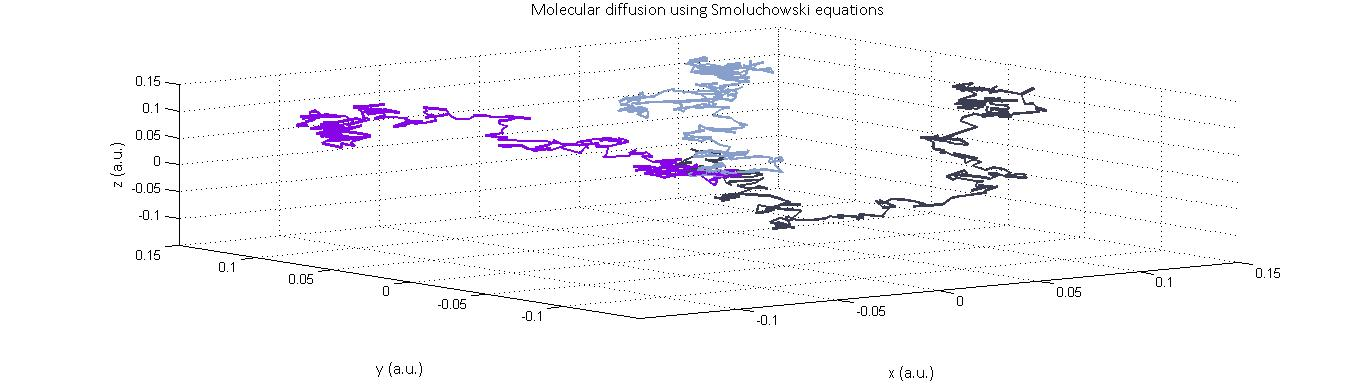# Plotted above are the trajectories of three molecules in 3-D diffusion according to the Smoluchowski equations.

Rate of collision within microcompartment modelsMicrocompartment/rate of collision models

# Modelling the effect of introducing a microcompartment on reaction rate

Our main approach to reducing the accumulation of toxic intermediates in the DCM degradation pathway is the expression of Pdu microcompartments in our bacteria that will contain high concentrations of both the DcmA enzyme and FdhA enzyme. In doing so, we increase the likelihood of a formaldehyde molecule produced by the action of DcmA encountering an FdhA enzyme before it leaves the microcompartment thus reducing the accumulation of formaldehyde.

To model the increased likelihood of reaction, we started with the Smoluchowski diffusion model above but discretized the system such that molecules could only occupy a fixed set of co-ordinates. This was done in order to define a reaction as occurring whenever two molecules occupied the same co-ordinate at the same time. While this does not necessarily ensure a reaction in the real system, we are assuming that collision rate is proportional to reaction rate and can therefore be used as an analogy.

Plotted here are two systems- one with complete freedom of molecular movement and one in which spatial constraints have been placed such that molecules which encounter the defined boundaries are reflected back into the system to represent the presence of the microcompartment: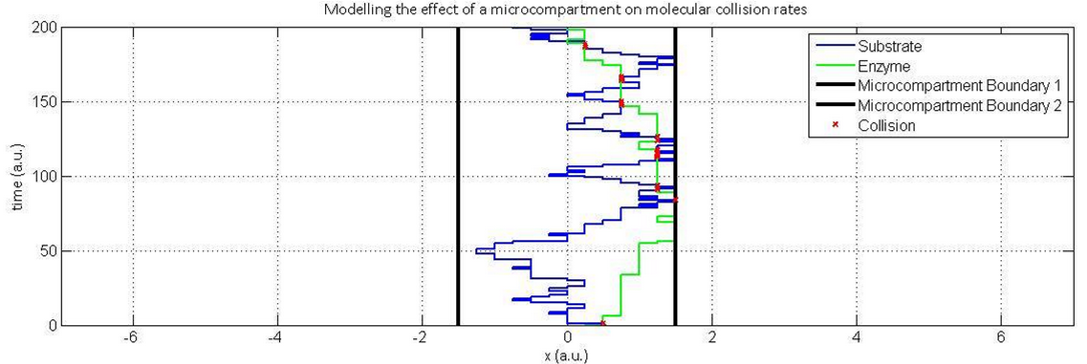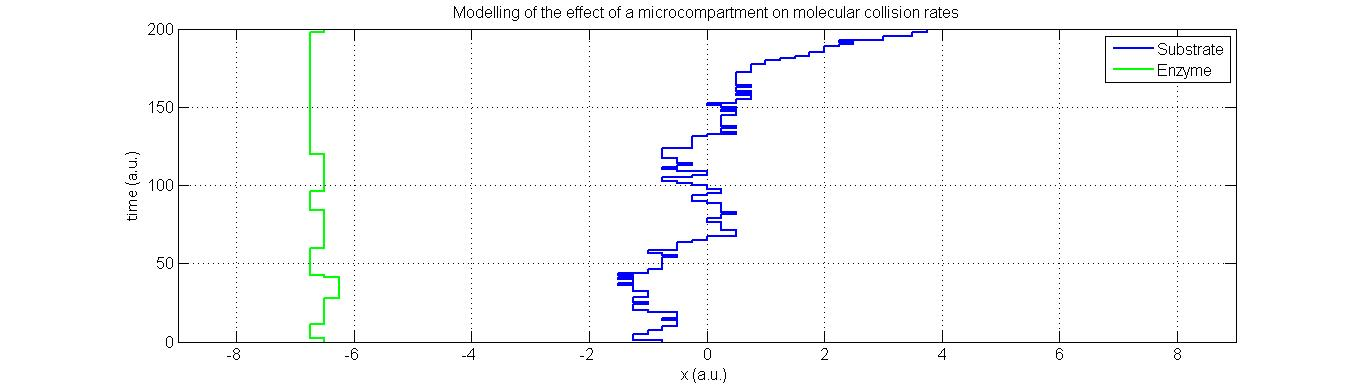In the models above, collisions are indicated through red marks. As suspected, the likelihood of a collision in a spatially constrained environment are far higher than in one where the molecules have complete freedom of movement. This is particularly true not only because of the region of movement allowed by the microcompartment, but also the initial distance between the enzyme and substrate at the point of substrate formation. Note also that the rate of diffusion of the enzyme, in green, has been made substantially lower than that of the formaldehyde- the far lighter and therefore more diffusive compound.

# 3D collision model

The 1-D collision model described above was quite easily expanded into a 3-D collision model that is more representative of the actual system. This results in simulations which appear far more realistic.

Illustrated above are the molecular trajectories of two different species- enzyme and substrate. The enzymes (red) are constrained to move within the microcompartment and are reflected at the microcompartment boundaries while the substrates (in blue) can freely diffuse anywhere in the system. While these simulations are very similar to the 1-D simulations previously described, they are far more computationally laborious.

Although the original plan was to run the simulation for several hundred enzymes and substrates and study the total number of collisions achieved, collisions are used as an analogy for reaction, it was quickly apparent that using this simulation to provide analytic results on the scales we wanted required far too much computation.

Given more time, access to more powerful computers and economization of our Matlab scripts, it would be possible to run this simulation for greater numbers of molecules than have been done thus far. This would then yield more data on the proportional increase in rate that results from introducing the microcompartment into the system.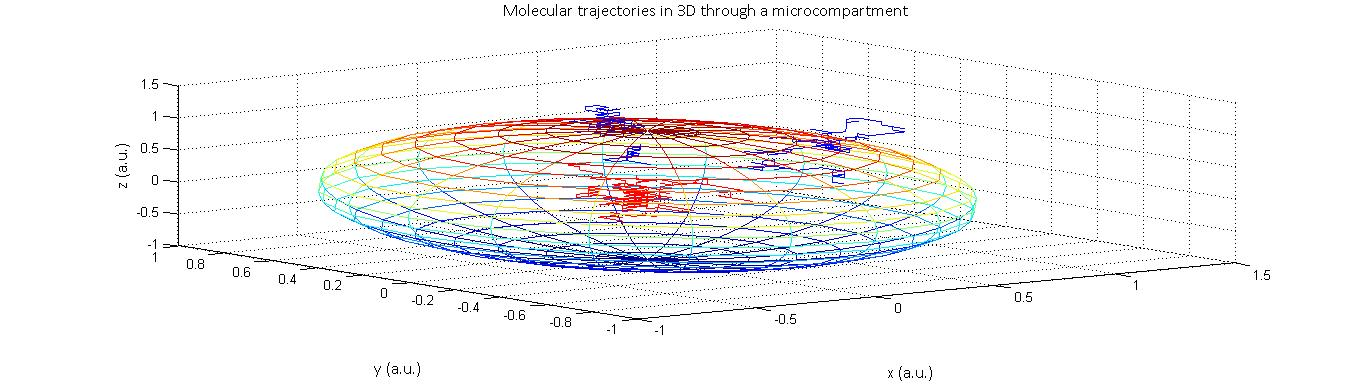# Illustrated above is an example of a 3-D stochastic diffusion model for a three substrate (blue) and two enzyme molecules (red).

Reducing accumulation of toxic intermediatesReducing accumulation of toxic intermediates
Formaldehyde is genotoxic because it forms protein-DNA and DNA-DNA cross-links. This interferes with DNA replication, repair, and transcription, as well as other processes. It has previously been reported that expression of dcmA in E. coli in the presence of DCM leads to cell death. We hypothesise to be caused by the formaldehyde that is released in the dcmA reaction. The localisation of dcmA into microcompartments means that the formaldehyde is also only locally produced within the microcompartment, thus preventing it from causing damage to DNA.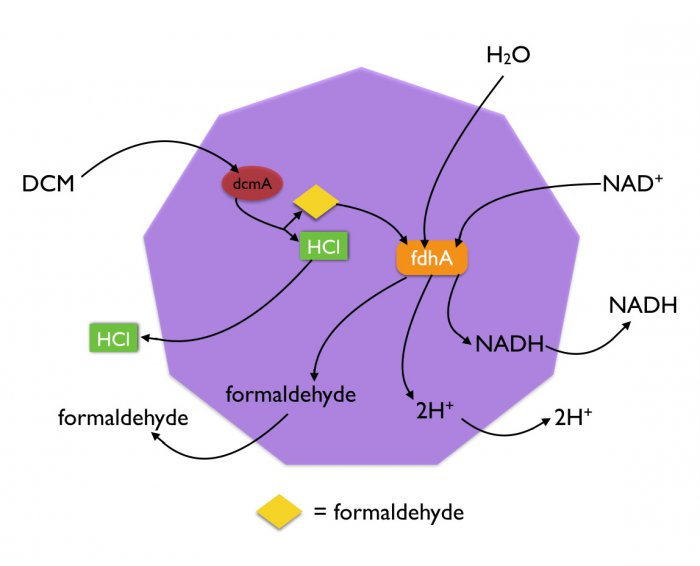Oxford iGEM 2014
To reduce the problem of formaldehyde toxicity in both E. coli and P. putida even more, we have expressed formaldehyde dehydrogenase (fdhA) in both strains. Using the 18-amino acid leader sequence, fdhA is co-localised to microcompartments along with dcmA. As the local concentration of fdhA is now also increased, the formaldehyde produced by dcmA is quickly broken down before it has a chance to damage the cell.
Another advantage of the co-localisation is that removal of one of the products of the dcmA reaction, formaldehyde, drives the reaction of dcmA forward. This further increases the rate of DCM breakdown in our system.
Modelling formaldehyde concentration against timeModelling formaldehyde concentration against time

# Predicted effect of microcompartments on formaldehyde concentration

Having deduced that microcompartments will increase the rate at which intermediate compounds are degraded, the next step was to create a simulation that would predict how microcompartments would therefore affect the concentration of formaldehyde molecules in the system. To do so, we created a 1-D simulation in which we started with a fixed number of molecules while constraining degradation and production of a species to within pre-defined spatial limits- representing the fact that both these phenomena can only occur in the microcompartment in our actual system.

In our system, the relative likelihood of degradation is far greater than that of production- this is done in order to ensure that accumulation of toxic compounds does not occur. The increased rate of degradation is the result of several factors:

1. Greater relative expression of FdhA than of DcmA- their expression ratios have been defined as approximately 2.5:1

2. Kcat in FdhA is substantially higher than in DcmA

3. The effect of the microcompartment will increase the relative likelihood of degradation of formaldehyde while leaving the rate of DCM degradation unchanged.

What our models suggest is that the microcompartments will not constrain the concentration of intermediates to be high only within the system. This is because the rate of diffusion of formaldehyde through the microcompartment barrier is unaffected, since its molecular size is much smaller than the pore size of the microcompartment. The significance of introducing the microcompartment is in fact to further increase the relative probability of degradation. This results in, as we expected, a net decrease in the total number of formaldehyde molecules, even when a high initial concentration is introduced, coupled with a decrease in concentration gradient throughout the system.

# Displayed below is one realization of a stochastic simulation (grey) alongside the deterministic response (red) of intermediate concentration at two points in time- one at 30 a.u. and the other at 200 a.u..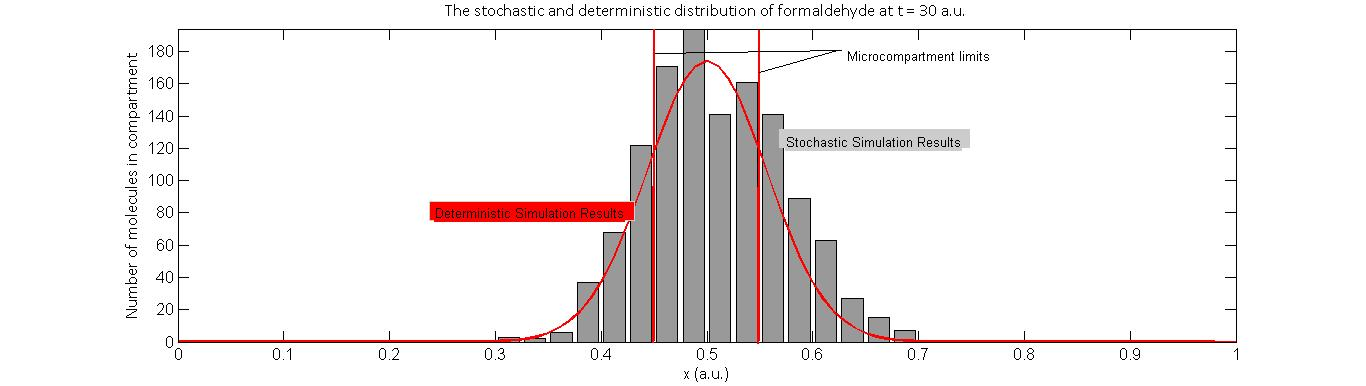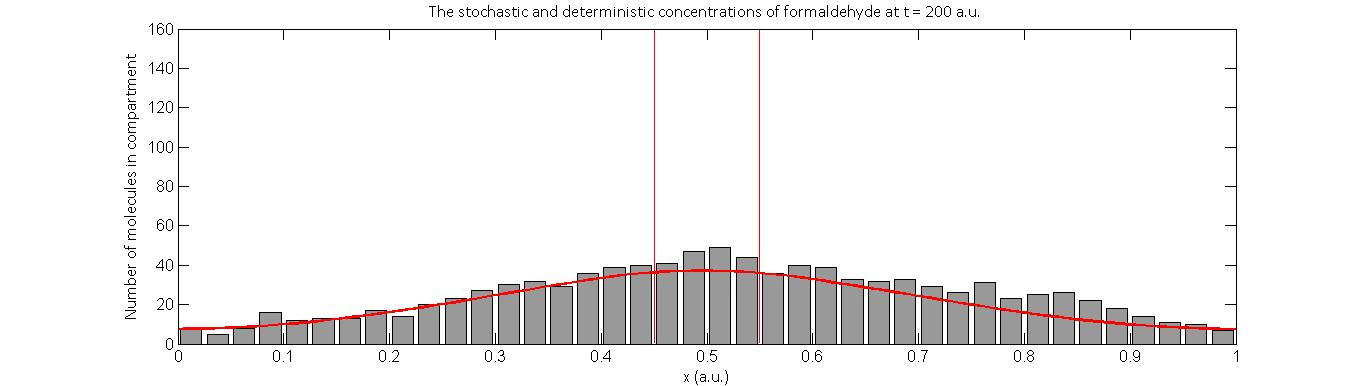Oxford iGEM 2014